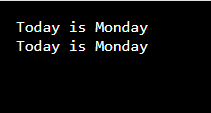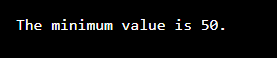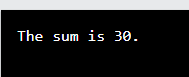# C++ Function

## Introduction to C++ Function

The C++ function is a code segment with a group of statements combined to perform a required task. For example, if we want to store a sum of two numbers, A and B, in a specific place. Now we will create a program in this way. First, we will add the given numbers and store that value in a home, C. Later on, wherever C is required, we can call that function instead of operating.

The main aim of creating a C++ function is to execute an action repetitively without writing the statements again. Write the required functions at the beginning of the program, and whenever essential, we can call the function to execute the job.

### Key Takeaways

• C++ functions are a collection of statements (the code) that collectively complete a job.
• Functions help to reduce code redundancy and keep the program modular and abstract.
• You can either use the C++ functions present in the library or design them according to your need.
• Every program has one main function, and every function has a return type. If a function doesn’t have any return type, it can be considered void.

### Types of C++ Function

Given below are the types of C++ function:

#### 1. Built-in Function

• The first is the Library function or the Built-in function.
• The C++ compiler package consists of these functions.
• In a software program, you can directly use them without creating the code.
• Some examples of such functions are sqrt(x), which returns the square root of a non-negative number ’x’; sin(x), which returns the sine of the angle x; and log(x), which returns the natural logarithm of the value x to the base e.

#### 2. User-Defined Function

• The user-defined function or the tailor-made function.
• You must create this function and add code to accomplish a specific task.
• Then, to use this function, you can call it from any location within your software program.
• For example, my_function( ), where you add certain code in the parentheses.

### Parts of Functions

Given below are parts of the functions:

#### 1. Declaration

• The function name is the specific name given to it.
• A parameter or an Argument consists of the required values of a function. Passing any value to a function at a certain point is unnecessary, so these become optional.
• Create a Parameter list by passing two or more values to a function where a comma separates each value.
• If it returns no value, the ’return type’ is ’void.’ Else you will have a return type. The return type, function name, and parameter list are combined to form the ’function header.’

#### 2. Definition

The function body consists of statements that execute an action.

The overall format of the function is:

``````void myFunction()  // declaration
// the body of the function (definition)
``````

### C++ Call Functions

• In a software program, you must call the function to transfer the program control to a function.
• You must type the function name followed by the parameter list to call a function.
• If the function returns a value, you must have a variable to store the returned value.
• You can call a function many times in a program.
• Herein, Monday is a user-defined function that prints the statement ’Today is Monday.’ The main() function calls the Monday function twice. So the statement ’Today is Monday.’ is printed twice as the output.

Code:

``````#include <iostream>
using namespace std;
void Monday()
cout << “Today is Mondayn”;

int main()
Monday();
Monday();
return 0;
``````

Output:There are two ways to call a function:

#### 1. Call by Value

• Before calling the function by its value, assign the value of an argument value to a parameter.
• But the values given to a parameter in called function will not impact the values of an argument in the calling function.

#### 2. Call by Reference

• Assign the address of an argument to the address of a parameter. In the called function, use the address of the argument to access it.
• The parameter’s value in the called function changes the argument’s value in the calling function.

### Examples of C++ Function

Given below are the examples mentioned:

#### Example #1

Code:

``````#include <iostream>
using namespace std;
int AB (int b1, int b2)

int b3;
if (b1 > b2)
b3 = b2;
else
b3 = b1;
return b3;

int main()

int a1 = 50;
int a2 = 100;
int a3;
a3 = AB (a1, a2);
cout  <<  “The minimum value is” <<  a3 <<”.”;
return 0;
``````

Output:#### Example #2

Code:

``````#include <iostream>
using namespace std;
int ADD (int m1, int m2)

int m3;
m3 = m1 + m2;
return m3;

int main()

int n1 = 10;
int n2 = 20;
int n3;
cout << “The sum is” << n3 << “.”;
return 0;
``````

Output:### Conclusion

C++ functions are a collection of code that accept specific values, work on these values, and return a value. Some functions are inbuilt, while users create others. The main idea behind creating the functions is to put the repeated codes under one heading and recall it when required. One cannot alter the values of the arguments in the called function.

### FAQs

Given below are the FAQs mentioned:

#### Q1. What are the C++ functions?

Answer: C++ functions are the statements that perform a required function. There are in-built functions as well as user-defined functions. You can define this function in a block at the beginning of your program and call them whenever required.

#### Q2. How many types of functions are there in C++?

Answer: C++ functions are of two kinds, Built-In or Library functions, and User-defined functions. Built-in functions are already available in C++, so you can grab them when needed. For example, min(), max(), swap(), gcd(), etc. In contrast, user-defined functions are customizable according to the user’s needs. For example, function_1 = (a + b) * (b + c); here, you perform this function, store the value in function_1, and call it in the main program.

#### Q3. Describe the main function in C++.

Answer: In C++, there will be at least one function and a mandatory function called the main function. Only the operating system can call the main function to execute the program written. A program can consist of numerous functions, but only one main function will exist.

#### Q4. Why do you need to use C++ functions?

Answer: The code that does the specific functionality is grouped and called a function. By using functions in C++, you can avoid repeating the codes. Also, if you need to change some code in the program, it is adequate to switch it on only one occasion in the function rather than throughout the program. Thus, the code is made modular.  The third purpose is an abstraction, which implies that you can leverage built-in functions (known as library functions) without focusing on their internal code. You can write the code inside a block that you consider a function. Hence you can call that function whenever you need it in the program. It helps to keep the program in a well-organized segment.

#### Q5. How can you call the C++ function?

Answer: The C++ functions can be called in two ways, call by value and call by reference. In the call-by-value method, only the variables defined in that function should pass while calling a function. When you call a function by the call-by-reference method, just the reference of an argument gets directed to complete the task.